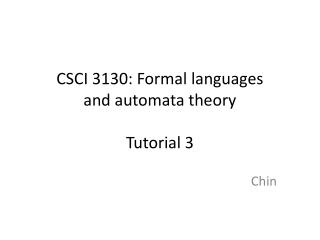Download PresentationCSCI 3130: Formal languages and automata theory Tutorial 3

# CSCI 3130: Formal languages and automata theory Tutorial 3 - PowerPoint PPT PresentationDownload Presentation## CSCI 3130: Formal languages and automata theory Tutorial 3

- - - - - - - - - - - - - - - - - - - - - - - - - - - E N D - - - - - - - - - - - - - - - - - - - - - - - - - - -
##### Presentation Transcript

1. Reminder • Homework 2 is due on next Monday.

2. Homework 1 • Problem 1 (c) Should state clearly what each state represents (d) Some of you accept the strings 02 & 20 in the DFA Some of you did not explain how the DFA works

3. Homework 1 • Problem 2 Be careful of e-transitions Many people (including myself!) missed the edge from q1 to q0 on input ‘0’ q1 -e-> q0 -0-> q1 -e-> q0 A few of you did not draw a transition table or a NFA without e-transitions, but the converted DFA is wrong. Do draw them to get partial credits.

4. Homework 1 • Problem 3 Most of you need to explain more clearly. Many of you just showed strings in L1 are also in L2, then conclude they are equivalent. What if some strings in L2 are not in L1? Do NOT do the following… L1 and L2 are the equivalent because L2 clearly represents the language of L1.

5. Closure Properties 1. If L is regular, is L’ = {wx: w ∈ L; x ∈ S*}also regular? 2. If L1 is not regular and L2 is not regular, is L1∩L2 also not regular?

6. Closure Properties 1. Yes. Let R be the regular expression of L. Then RS* is also a regular expression. Hence regular. 2. No. L1 = 0n1n, L2 = 1n0n. Then L1 and L2 are both non-regular. But L1∩L2 = {e}, which is regular.

7. Non regularity • For every n, choose onez of length ≥ n in L, such that for every way of writing z = u v w where • |uv| ≤ n and • |v| ≥ 1, • the string u vi w is not in L for some i≥ 0. • z depends on n • ucan be empty string • i can be 0 (very useful) • You only have to choose one z

8. Pumping lemma • What’s wrong in the proof? L = {11111} is not regular. Proof: Suppose n = 2 and z = 11111, which is in L. Write z = uvw, where |uv| ≤ 2 and |v|≥ 1. Then uv = 1 or uv = 11. If uv = 1, then uv2w = 11 1111 is not in L. If uv = 11 and u = 1, then uv2w = 1 11 111 is not in L. If uv = 11 and u = e, then uv2w = 1111 1111 is not in L. Therefore, L is not regular.

9. Pumping lemma • For every nthere exists z of length ≥ n in L, such that for every wayof writing z = u v w where • n is not fixed.

10. Pumping lemma • What’s wrong in the proof? L = {x: x = 1k0n1n,k≥ 0, n ≥ 0} is not regular. Proof: Suppose the DFA has n states. Then z = 1110n1n is in L. Write z = uvw, where u = 111, v = 0n-3, w = 031n Then |uv| ≤ n and |v|≥ 1. But uv0w = 111031n is not in L. Therefore, L is not regular.

11. Pumping lemma • For every nthere exists z of length ≥ n in L, such that for every way of writing z = u v w where • |uv| ≤ n and • |v| ≥ 1, the string u vi w is not in L for some i≥ 0. • v = 0n-3 • n-3 can be less than 1. • Write z = uvw, where u = 111, v = 0n-3, w = 031n. • You cannot decide what u, v, w are.

12. Pumping lemma Template Suppose L is regular and its DFA has n states. Then z = _______ (z should contain n somewhere) is in L. Write z = uvw, where |uv| ≤ n and |v|≥ 1. Argue no matter how we write z = uvw, the string uv_w is not in L(choose i in _, i can be 0) Therefore L is not regular.

13. Pumping lemma • Are the following regular? • S = {a, b, c} L1 = {w: w has the same number of patterns ab and ba} L2 = {aibj : i < j}

14. Pumping lemma 1. Yes. (covered in lecture?) 2. No. Suppose L2 is regular and its DFA has n states. Then z = anbn+1 is in L. Write z = uvw, where |uv| ≤ n and |v|≥ 1. Since|uv| ≤ n, uv contains ‘a’sonly, meaningv contains ‘a’s only. Since |v|≥ 1, v must contain at least 1 a. Then the number of ‘a’s in uv2w is n + |v| ≥ n + 1, which is the number of b in the string. Therefore it is not in L and so L is not regular.

15. Homework 2 • Questions? • Want hints?

16. End • Any questions?